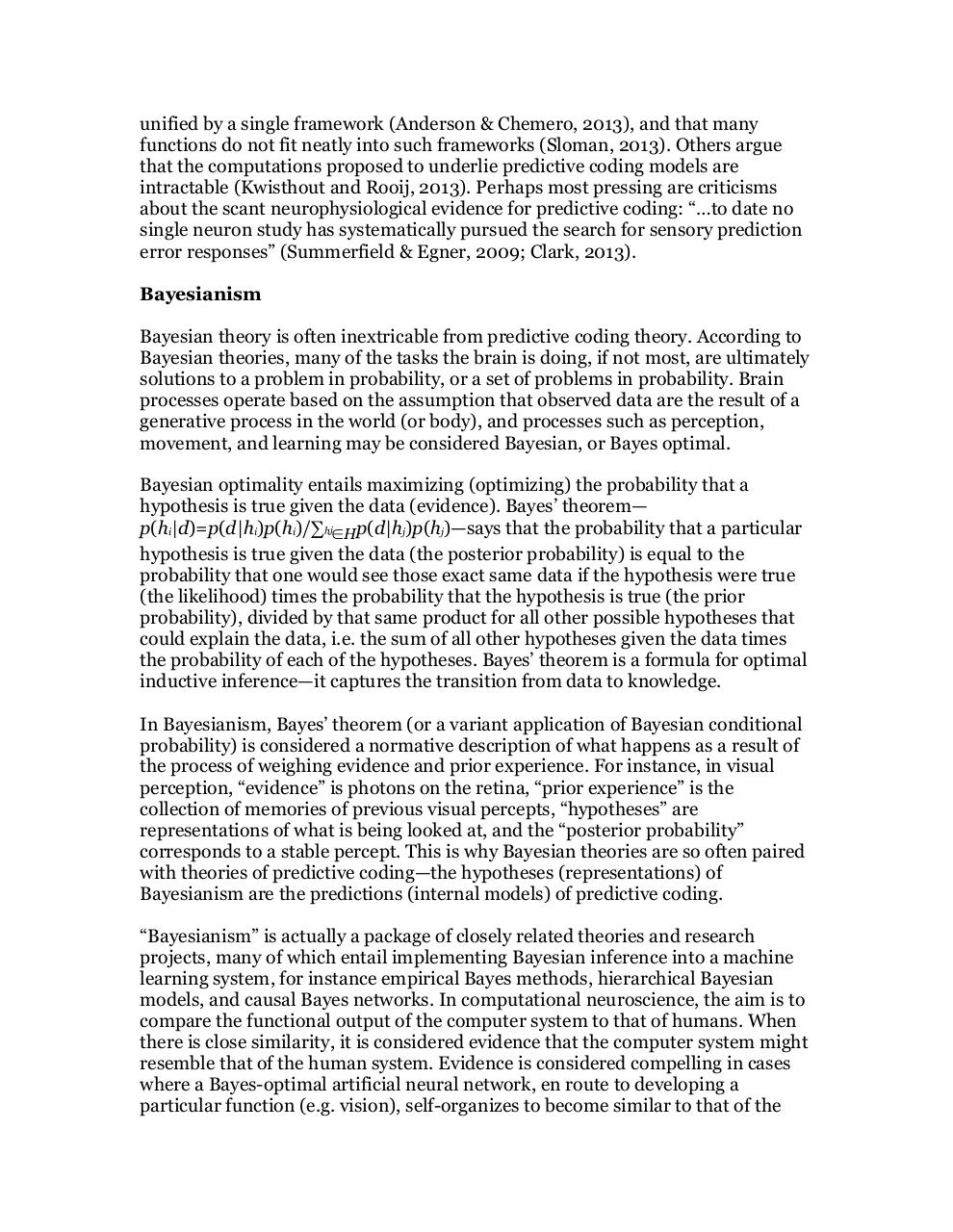# PDF Archive

Easily share your PDF documents with your contacts, on the Web and Social Networks.

## Probability and Cognition.pdfPage 1 2 34516

#### Text preview

unified by a single framework (Anderson &amp; Chemero, 2013), and that many
functions do not fit neatly into such frameworks (Sloman, 2013). Others argue
that the computations proposed to underlie predictive coding models are
intractable (Kwisthout and Rooij, 2013). Perhaps most pressing are criticisms
about the scant neurophysiological evidence for predictive coding: “…to date no
single neuron study has systematically pursued the search for sensory prediction
error responses” (Summerfield &amp; Egner, 2009; Clark, 2013).
Bayesianism
Bayesian theory is often inextricable from predictive coding theory. According to
Bayesian theories, many of the tasks the brain is doing, if not most, are ultimately
solutions to a problem in probability, or a set of problems in probability. Brain
processes operate based on the assumption that observed data are the result of a
generative process in the world (or body), and processes such as perception,
movement, and learning may be considered Bayesian, or Bayes optimal.
Bayesian optimality entails maximizing (optimizing) the probability that a
hypothesis is true given the data (evidence). Bayes’ theorem—
p(hi|d)=p(d|hi)p(hi)/∑hj∈Hp(d|hj)p(hj)—says that the probability that a particular
hypothesis is true given the data (the posterior probability) is equal to the
probability that one would see those exact same data if the hypothesis were true
(the likelihood) times the probability that the hypothesis is true (the prior
probability), divided by that same product for all other possible hypotheses that
could explain the data, i.e. the sum of all other hypotheses given the data times
the probability of each of the hypotheses. Bayes’ theorem is a formula for optimal
inductive inference—it captures the transition from data to knowledge.
In Bayesianism, Bayes’ theorem (or a variant application of Bayesian conditional
probability) is considered a normative description of what happens as a result of
the process of weighing evidence and prior experience. For instance, in visual
perception, “evidence” is photons on the retina, “prior experience” is the
collection of memories of previous visual percepts, “hypotheses” are
representations of what is being looked at, and the “posterior probability”
corresponds to a stable percept. This is why Bayesian theories are so often paired
with theories of predictive coding—the hypotheses (representations) of
Bayesianism are the predictions (internal models) of predictive coding.
“Bayesianism” is actually a package of closely related theories and research
projects, many of which entail implementing Bayesian inference into a machine
learning system, for instance empirical Bayes methods, hierarchical Bayesian
models, and causal Bayes networks. In computational neuroscience, the aim is to
compare the functional output of the computer system to that of humans. When
there is close similarity, it is considered evidence that the computer system might
resemble that of the human system. Evidence is considered compelling in cases
where a Bayes-optimal artificial neural network, en route to developing a
particular function (e.g. vision), self-organizes to become similar to that of the